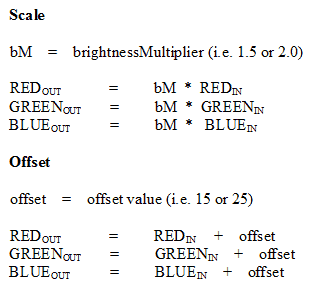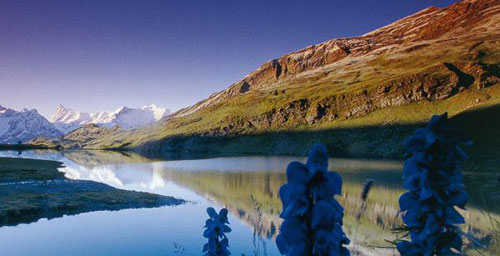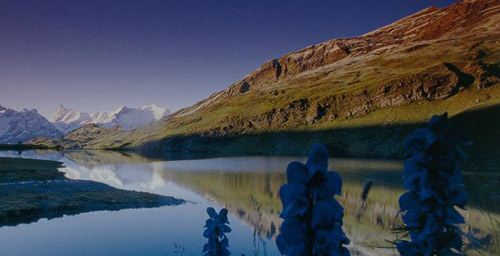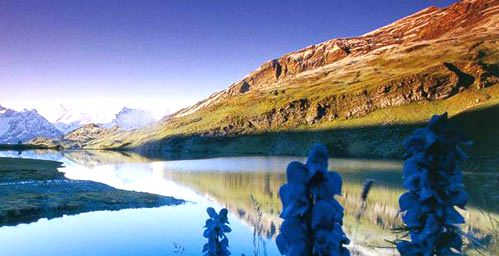# Changing brightness of an image in html5 canvas.

Sometimes we need to adjust the brightness of a photo. It can easily be done using some image editor programs. But, if you want to adjust the brightness using the JavaScript canvas api, follow this tutorial, and see how easily it can be achieved.

The steps

1) Load the image, and draw it inside the canvas.

2) Get the pixel data of the image. It is needed to access color channels(RGB) values.

3) For every pixel scale or offset all the colors equally.

Adjusting brightness using the scaling method is achieved by multiplying all the three colors(RGB) by a number. To increase brightness, multiply by a number greater than 1. To decrease, multiply by a number less than 1 and greater than 0.

Another way to control the brightness is by using the offset method. You can offset the colors by adding a number to all the colors. You might have guessed that adding a positive number increases the brightness, whereas a negative number decreases it.

4) Put the image data in the canvas.

In this tutorial, we will apply the multiplying(scaling) method.

OverviewThe code snippet:

Download the attachment containing the full source at the end of this tutorial.

var iD = context.getImageData(imgX, imgY, img.width, img.height);
var dA = iD.data; // raw pixel data in array

var brightnessMul = 1.35; // brightness multiplier

for(var i = 0; i < dA.length; i += 4)
{
var red = dA[i]; // Extract original red color [0 to 255]
var green = dA[i + 1]; // Extract green
var blue = dA[i + 2]; // Extract blue

brightenedRed = brightnessMul * red;
brightenedGreen = brightnessMul * green;
brightenedBlue = brightnessMul * blue;

/**
*
* Remember, you should make sure the values brightenedRed,
* brightenedGreen, and brightenedBlue are between
* 0 and 255. You can do this by using
* Math.max(0, Math.min(255, brightenedRed))
*
*/

dA[i] = brightenedRed;
dA[i + 1] = brightenedGreen;
dA[i + 2] = brightenedBlue;
}

context.putImageData(iD, imgX, imgY);

Original imageBrightness Multiplier = 0.75 (decreased)Brightness Multiplier = 1.35 (increased)Attachment:html source code and photo filesYou might want to contribute to Qoncious.com: Option 1 \$2.00 USDOption 2 \$5.00 USDOption 3 \$10.00 USD